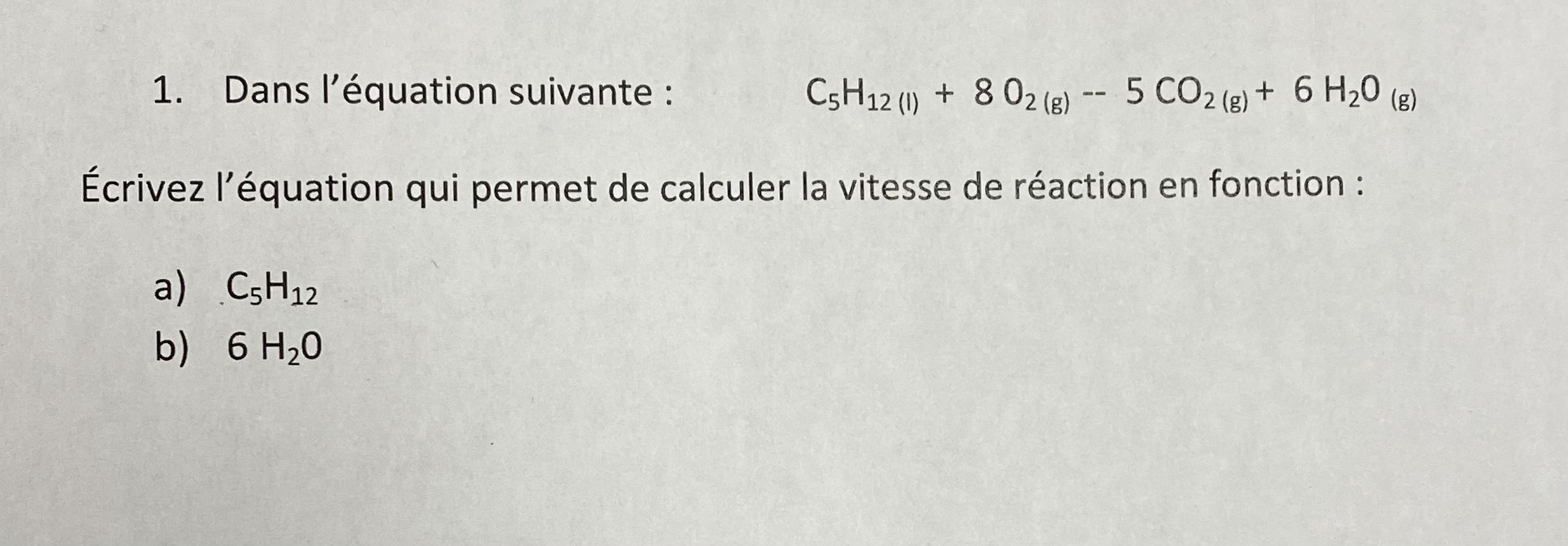# Help Zone

### Student QuestionThis is my chemistry question. Can someone pls help me.

Chemistry## Explanations (1)

•Explanation from AlloprofExplanation from Alloprof

This Explanation was submitted by a member of the Alloprof team.

Options
Team Alloprof • 1yr. edited March 2022

The question asks you to calculate the reaction rate as a function of one of the reactants or one of the products. The question isn't specific as to what type of equation is needed, so an answer like the following is acceptable:

$$r_{C_5H_{12}} = -\frac{[C_5H_{12}]}{∆t}$$

Please note that the - is included in the equation because the concentration of pentane decreases.

$$r_{H_2O} = \frac{[H_2O]}{∆t}$$

Don't hesitate to ask for more help if you need it!